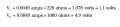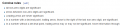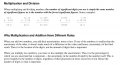# The double squiggle symbol ≈ how did I get this 1.1

#### Manu1

Joined Mar 30, 2016
23
Hello guys here I am posting my confusion, I am learning electronics and just entered toThe double squiggle symbol≈ and in this math I got 1.078 results how ever ≈ this equation is for round off the results, but I am not getting how they did this round off from 1.078 to just 1.1?
Note : I am poor in math and beginner in electronics don't show your talent here if you do I didn't understand a single thing be a beginner with a beginner#### bertus

Joined Apr 5, 2008
22,141
•Manu1

Joined Jul 18, 2013
27,012
Do you know what round off or round up means or how it is done?
The exact mathematical answer is obviously 1.078, but in some areas of operation, an accuracy to 3 places of decimals is not required, hence the round up/down.
Max.

•Manu1

#### #12

Joined Nov 30, 2010
18,224
This isn't electronics. It's basic math. "How to round off numbers."
If you try to measure 1.078 volts with an analog meter, it's just about impossible to see the difference between 1.078 and 1.08
What the heck. Let's just call it 1.1, give or take 2 or 3 %.
See where I'm going? If the measurement is too small to see, it's too small to care about.
Most of the time, you don't even buy parts that are accurate to 2%, so the measurement in a real circuit is only as good as the quality of the parts.
In a mathematical sense, it is not even valid to pretend you have a 4 digit measurement when you're using 5% resistors.
You have to be on my level, doing precision analog design, to even care about a 2% difference.

•Manu1

#### Manu1

Joined Mar 30, 2016
23
Do you know what round off or round up means or how it is done?
The exact mathematical answer is obviously 1.078, but in some areas of operation, an accuracy to 3 places of decimals is not required, hence the round up/down.
Max.
Yes I don't know how it is done

#### jpanhalt

Joined Jan 18, 2008
11,087
There is an added reason to "round" the result. Consider the number of "significant figures" for the values being used (https://en.wikipedia.org/wiki/Significant_figures ). Thus, although the calculated result may have any number of digits, the ones that are significant are limited in number.For example, in your first equation, "0.0049" has how many significant figures? Whatever that answer is limits the number of significant figures the answer can have. Albeit, people don't always follow that rule for perceived practical reasons.

John

•Manu1

#### Manu1

Joined Mar 30, 2016
23
Hey thank you all, but instead of writing here can anyone do paper work and upload? So this way will be very helpful to understand.. Thank you again

#### SLK001

Joined Nov 29, 2011
1,548
Two significant digits times two significant digits will correctly have an answer that is two significant digits.

#### Manu1

Joined Mar 30, 2016
23
Two significant digits times two significant digits will correctly have an answer that is two significant digits.#### #12

Joined Nov 30, 2010
18,224
I think what you are saying is that you can't understand why math can arrive at an answer which has digits that have no significant value.
Try this: Suppose you measure a circle on a piece of paper and find that it is 2 inches in diameter, as close as you can tell.
Circumference = Pi times the diameter.
multiply 2 times Pi
The answer starts out 6.283185307 and goes on forever.
Do you really think you can measure a circle accurately to 9 decimal places?
Can you cut a piece of paper to fit against the circle, accurate to 9 decimal places?

•Manu1

#### wayneh

Joined Sep 9, 2010
17,267
Maybe it's worth noting that mathematics is a human construct, an ideal. I once had a math exam question that could be answered numerically, say with 42. I was marked down for doing that, because the professor wanted the symbolic answer. To his way of thinking, using an actual number somehow dirtied the perfection.

Anyone that has worked in the real world knows that a calculated value with a long string of digits is absurd. You can't measure anything that precisely, and your accuracy may be off as well. Two significant figures is typical and more than three starts becoming very difficult.

There's a whole science of how to maintain proper significant figures when adding, multiplying, and averaging numbers. Any introductory text on analytical chemistry would cover it, and I'm sure you could find it in other sources as well.

The about symbol is used to explicitly note that a value has been rounded or approximated for practical reasons. For instance you might write that 3 x 3.3 is about 10. You wouldn't use the equals sign because it would not be technically correct.

#### GopherT

Joined Nov 23, 2012
8,009
....question that could be answered numerically, say with 42.
And, of all numbers you just happened to pick 42 at random.

•djsfantasi and wayneh

#### wayneh

Joined Sep 9, 2010
17,267
That's honestly my recollection of it! After a couple glasses of tequila.#### #12

Joined Nov 30, 2010
18,224
To his way of thinking, using an actual number somehow dirtied the perfection.
I also had a nasty math teacher. I think the answer was x + 1/x
He marked it wrong because I wrote the x in the fraction the same size as the x standing alone and, "There are no capital X's in that equation".
Point is, he wasn't grading my understanding of the math, he was grading some pedantic belief he had.

#### WBahn

Joined Mar 31, 2012
28,475
Two significant digits times two significant digits will correctly have an answer that is two significant digits.
EDIT: @SLK001 You were correct, I was wrong. Had a brain fart. Thanks to @GopherT for pointing it out to me.

Wrong.

The number of sig figs produced by multiplication is the sum of the number of sig figs in the factors.

Just consider an example:

73 x 79 = 5767

Your statement would require that this be rounded to 5800.

Last edited:

#### GopherT

Joined Nov 23, 2012
8,009
Wrong.

The number of sig figs produced by multiplication is the sum of the number of sig figs in the factors.

Just consider an example:

73 x 79 = 5767

Your statement would require that this be rounded to 5800.

What? 5800 is the correct answer, assuming there really us uncertainty in 73 and 79 (that is, you were not counting potatoes (73), and then counting the number of eyes on each potato (which happened to be exactly 79 on every potato). Then there is no uncertainty and you actually have an infinite number of significant figures.

If there is uncertainty in each of the two numbers and the best you can report is 73 and 79, then 5800 is the right answer. Here is how I've lived my life, read in textbooks and taught students...#### WBahn

Joined Mar 31, 2012
28,475
What? 5800 is the correct answer, assuming there really us uncertainty in 73 and 79 (that is, you were not counting potatoes (73), and then counting the number of eyes on each potato (which happened to be exactly 79 on every potato). Then there is no uncertainty and you actually have an infinite number of significant figures.

If there is uncertainty in each of the two numbers and the best you can report is 73 and 79, then 5800 is the right answer. Here is how I've lived my life, read in textbooks and taught students...

View attachment 103617
Yeah, brain fart on my part.

The best way to show it is to propagate the uncertainties.

When we say that 73 is good to 2 sig figs and don't give any more info than that, the best we can assume is that it is know to 1/2 of the least significant digit.

So, without getting into the math, the product of the two is

z = x·y = (73 ± 0.5)(79 ± 0.5)

z = 5767 ± 39.5 ± 36.5 + 0.25

While the last three terms are quasi-independent, so we combined them as Pythagorean elements getting

z = 5767 ± 53.8

So we are only justified two sig figs.

Looking at it more rigorously and assuming the errors in x and y are normally distributed and independent:

$$dz \; = \; \sqrt{ {\( \frac{\partial z}{\partial x}$$}^2 dx \; + {$$\frac{\partial z}{\partial y}$$}^2 dy}
\)

If

$$z \; = \; x \; + \; y$$

then

$$dz \; = \; \sqrt{ {\( y \: dx$$}^2 \; + {$$x \: dy$$}^2}
\)

$$dz \; = \; \sqrt{ {\( \frac{z}{x} \: dx$$}^2 \; + {$$\frac{z}{y} \: dy$$}^2}
\)

$$\frac{dz}{z} \; = \; \sqrt{ {\( \frac{dx}{x}$$}^2 \; + {$$\frac{dy}{y}$$}^2}
\)

If the uncertainty, as a fraction, is the same for x and y, then we have

$$\frac{dz}{z} \; = \; \sqrt{2} \frac{dx}{x} \; = \; \sqrt{2} \frac{dy}{y}$$

while if one uncertainty, say dx/x, is much larger than the other, we have

$$\frac{dz}{z} \; \approx \; \frac{dx}{x}$$

So we have, at best, the number of sig figs in the least certain number and, at worst, 70% of the sig figs of either when the certainty is comparable.

•GopherT

#### SLK001

Joined Nov 29, 2011
1,548
EDIT: @SLK001 You were correct, I was wrong. Had a brain fart. Thanks to @GopherT for pointing it out to me.

Wrong.

The number of sig figs produced by multiplication is the sum of the number of sig figs in the factors.

Just consider an example:

73 x 79 = 5767

Your statement would require that this be rounded to 5800.
Hey! I'm not always wrong!

•Sinus23 and absf